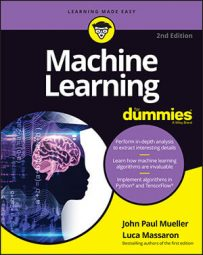##### Machine Learning For DummiesIdeally, in machine learning you can get the best results when your features don’t completely correlate with each other and each one has some predictive power with respect to the response you’re modeling. In reality, your features often do correlate with each other, displaying a high degree of redundancy in the information available to the dataset.

Having redundant data means that the same information is spread across multiple features. If it’s exactly the same information, it represents a perfect collinearity. If, instead, it’s not exactly the same information but varies in some way, you have collinearity between two variables or multicollinearity between more than two variables.

Redundant data is a problem that statistical theory created solutions to address long ago (because statistical computations can suffer a lot from multicollinearity). You might consider the topic under a statistical point of view, illustrating using the concepts of variance, covariance, and correlation. You can imagine each feature as bearing different informative components, mixed in different proportions:

• Unique variance: The redundancy is unique to a particular feature, and when correlated or associated with the response, it can add a direct contribution in the prediction of the response itself.
• Shared variance: The redundancy is common with other features because of a causality relationship between them. In this case, if the shared information is relevant to the response, the learning algorithm will have a difficult time choosing which feature to pick up. And when a feature is picked up for its shared variance, it also brings along its specific random noise.
• Random noise component: Information due to measurement problems or randomness that isn’t helpful in mapping the response but that sometimes, by mere chance (yes, luck or misfortune is part of being random), can appear related to the response itself.
Unique variance, shared variance, and random noise fuse together and can’t be separated easily. Using feature selection, you reduce the impact of noise by selecting the minimum set of features that work best with your machine learning algorithm. Another possible approach is based on the idea that you can fuse that redundant information together using a weighted average, thus creating a new feature whose main component is the shared variance of multiple features, and its noise is an average of previous noise and unique variance.

For instance, if A, B, and C share the same variance, by employing compression you can obtain a component (so it is called a new feature) made up of the weighted summation of the three features such as 0.5*A+0.3*B+0.2*C. You decide the weights on the basis of a particular technique called singular value decomposition (SVD).

SVD has various applications, not just in compressing data but also in finding latent factors (hidden features in our data) and in recommender systems, which are systems for discovering what someone might like in terms of products or films based on previous selections. For compression purposes, you might consider a technique called principal components analysis (PCA), which uses parts of the SVD outputs.

PCA works simply and straightforwardly: It takes as an input a dataset and returns a new, reconstructed dataset of the same shape. In this new dataset, all the features, called components, are uncorrelated, and the most informative components appear at the beginning of the dataset.

PCA also offers a report of how each component equates to the initial dataset. By summing the informative value of the new components, you may find that a few components express 90 percent or even 95 percent of the original information. Taking just those few components is equivalent to using the original data, thus achieving a compression of your data by removing redundancies and reducing the number of features.

As an example, the following example refers to the Boston dataset and uses Python’s Scikit implementation of PCA. R has many equivalent functions, the most popular being princomp, which you can learn about by using the help(princomp) command to obtain more information and some examples of its usage. Here is the Python code snippet for testing the effectiveness of a PCA:

`from sklearn.datasets import load_boston`

`from sklearn.decomposition import PCA`

`from sklearn.preprocessing import scale`

`import numpy as np`

`from sklearn.datasets import load_boston`

`from sklearn.decomposition import PCA`

`from sklearn.preprocessing import scale`

`import numpy as np`

`boston = load_boston()`

`X, y = boston.data, boston.target`

`pca = PCA().fit(X)`

After calculating the PCA, the example proceeds to print the informative power of this new reconstructed dataset:

`print (' '.join(['%5i'%(k+1) for k in range(13)]))`

`print (' '.join(['-----']*13))`

`print (' '.join(["%0.3f" % (variance) for variance`

`in pca.explained_variance_ratio_]))`

`print (' '.join(["%0.3f" % (variance) for variance`

`in np.cumsum(pca.explained_variance_ratio_)]))`

` `

`1 2 3 4 5 6 7 8 9 ...`

`----- ----- ----- ----- ----- ----- ----- ----- ----- ...`

`0.806 0.163 0.021 0.007 0.001 0.001 0.000 0.000 0.000 ...`

`0.806 0.969 0.990 0.997 0.998 0.999 1.000 1.000 1.000 ...`

In the printed report, the thirteen components account for a cumulative dataset that exceeds 85 percent of the original when taking into account six components out of 13 and 95 percent with nine components. Using a reconstructed dataset with fewer components than the number of the original features often proves beneficial to the machine learning processes by reducing memory usage and computation time and by containing the variance of the estimates, thus assuring the stability of the results.